# Telangana SSC Board (TS SSC) Question Paper 1 for Class 10th Maths 2018 In PDF

## Telangana 10th Annual Exam Question Papers 2018 Maths Paper 1 with Solutions – Free Download

Telangana SSC Question Paper Maths Paper 1 with solutions is available at BYJU’S with detailed explanations along with the required formulas and graphs (or images) so that class 10 students can understand them easily.

BYJU’S provided all the previous years question papers of the Telangana SSC board with two papers each having part A and part B. TS SSC Board Question Paper 1 for Class 10th Maths 2018 can be downloaded by the students in a pdf form and practice them at any time. Also, they are provided with solutions to help the students in referring to the accurate solutions.

### Telangana Board SSC Class 10 Maths 2018 Question Paper 1 with Solutions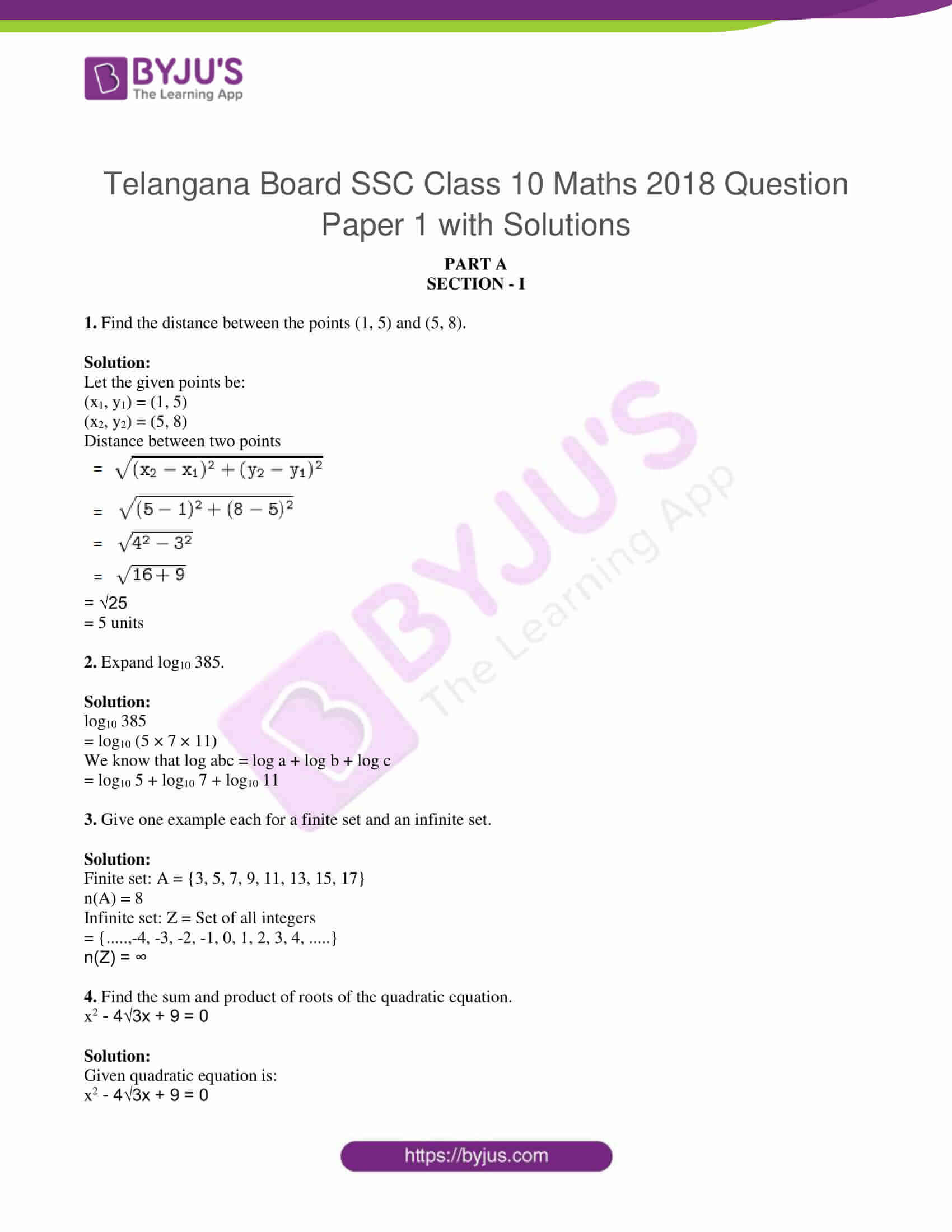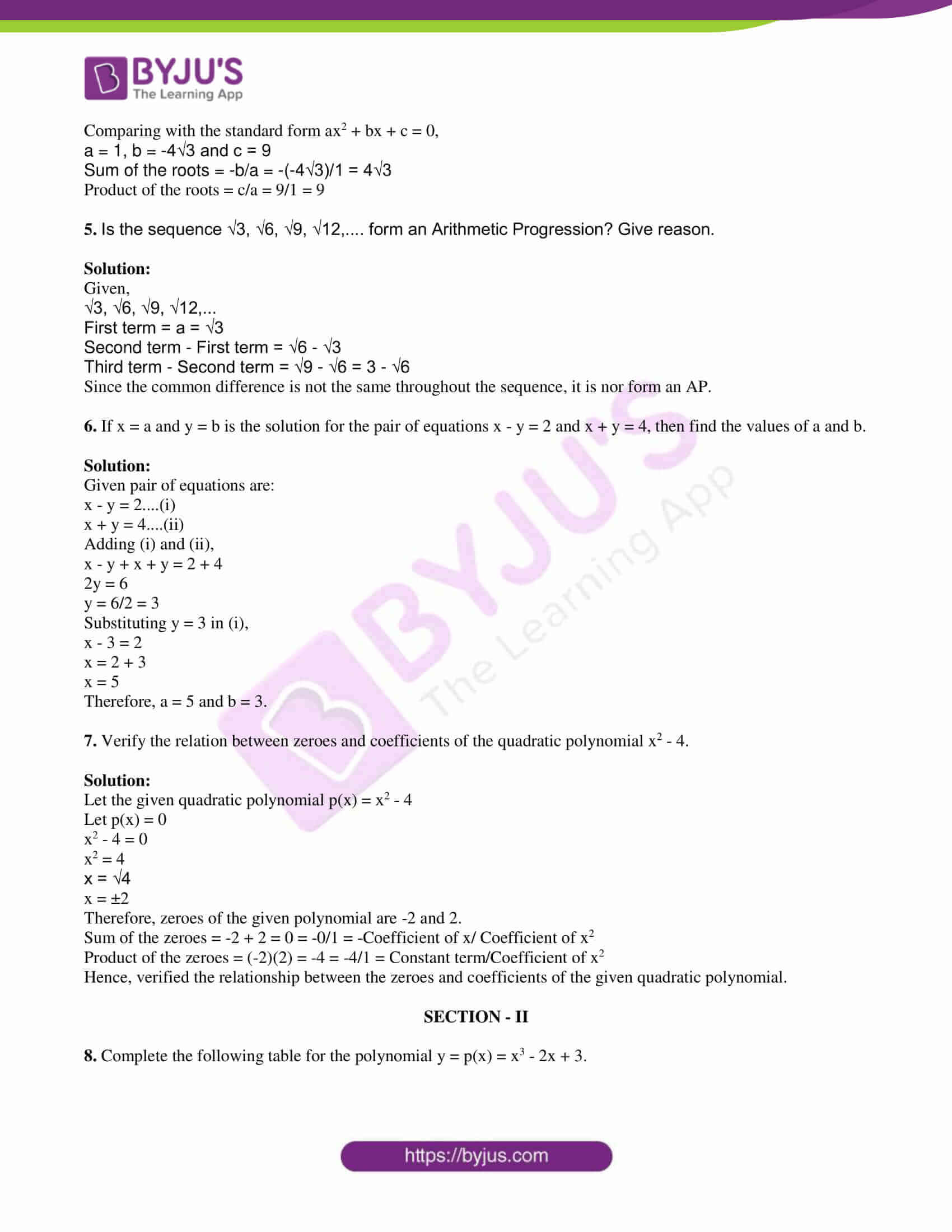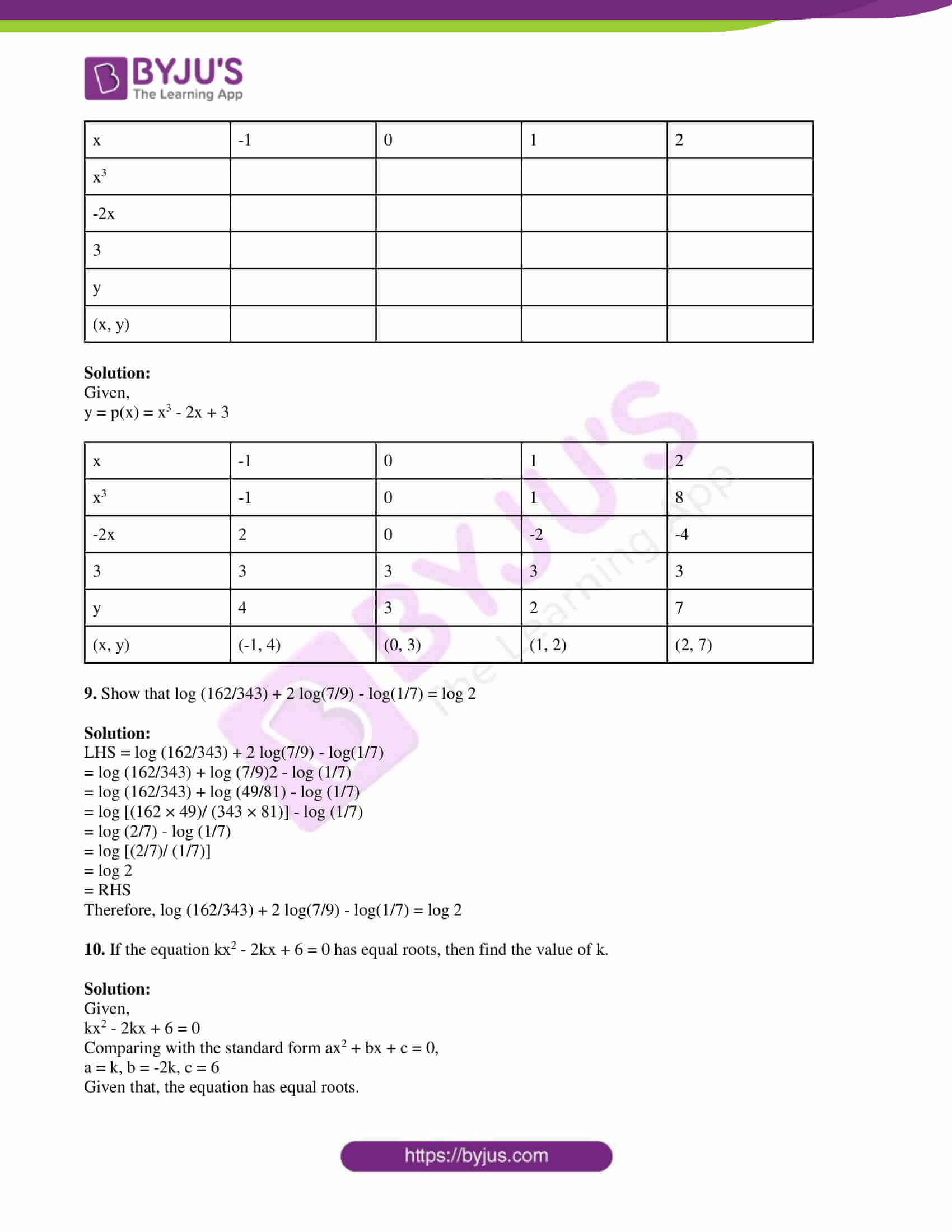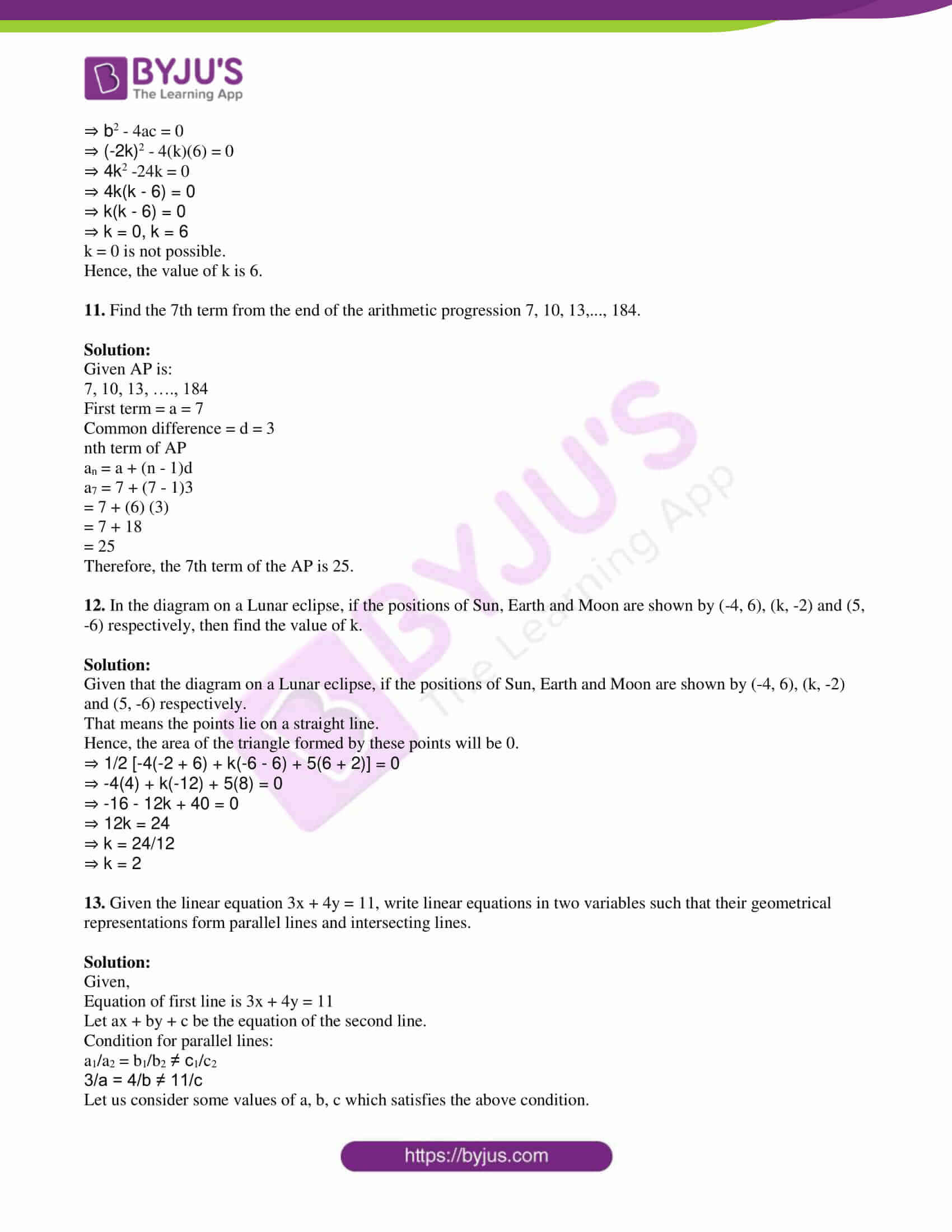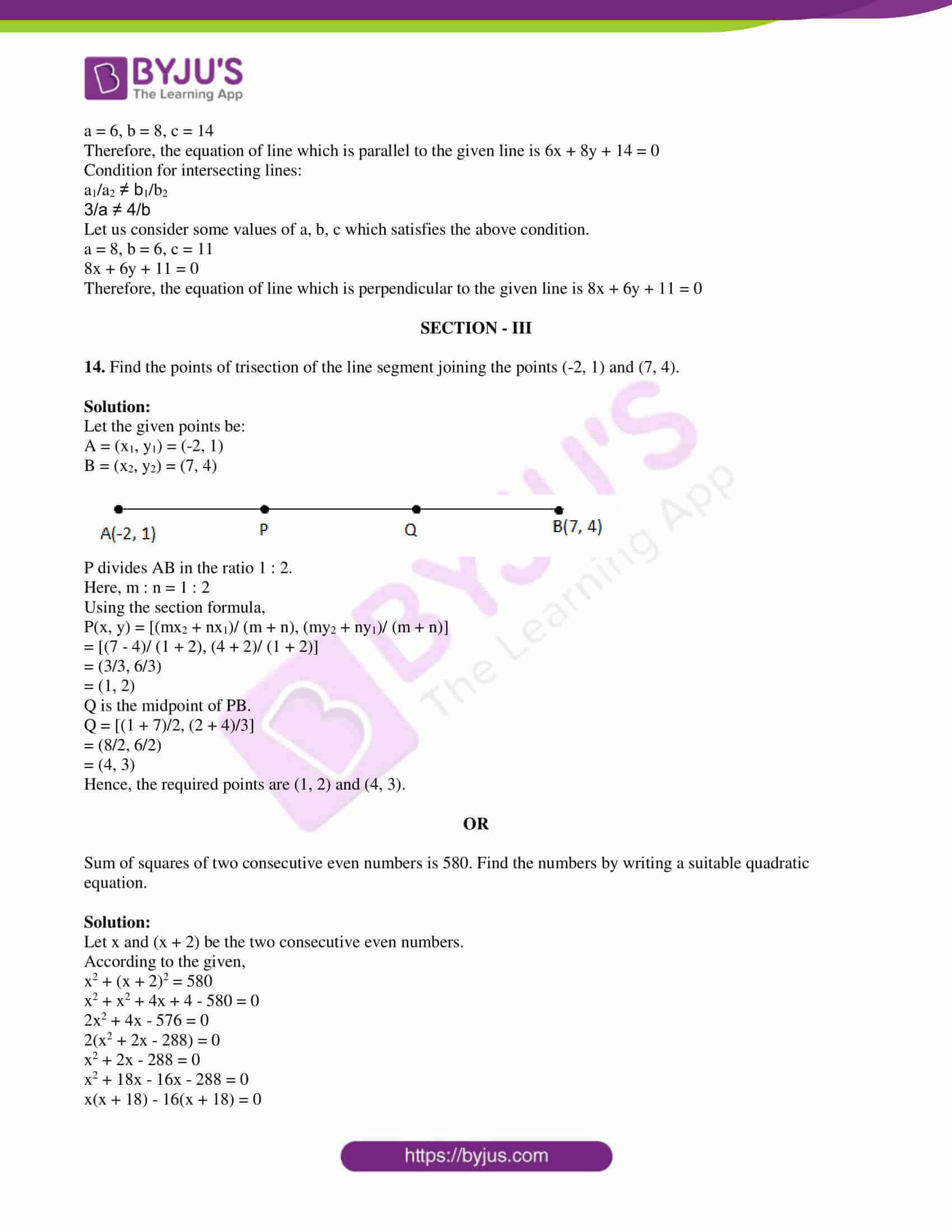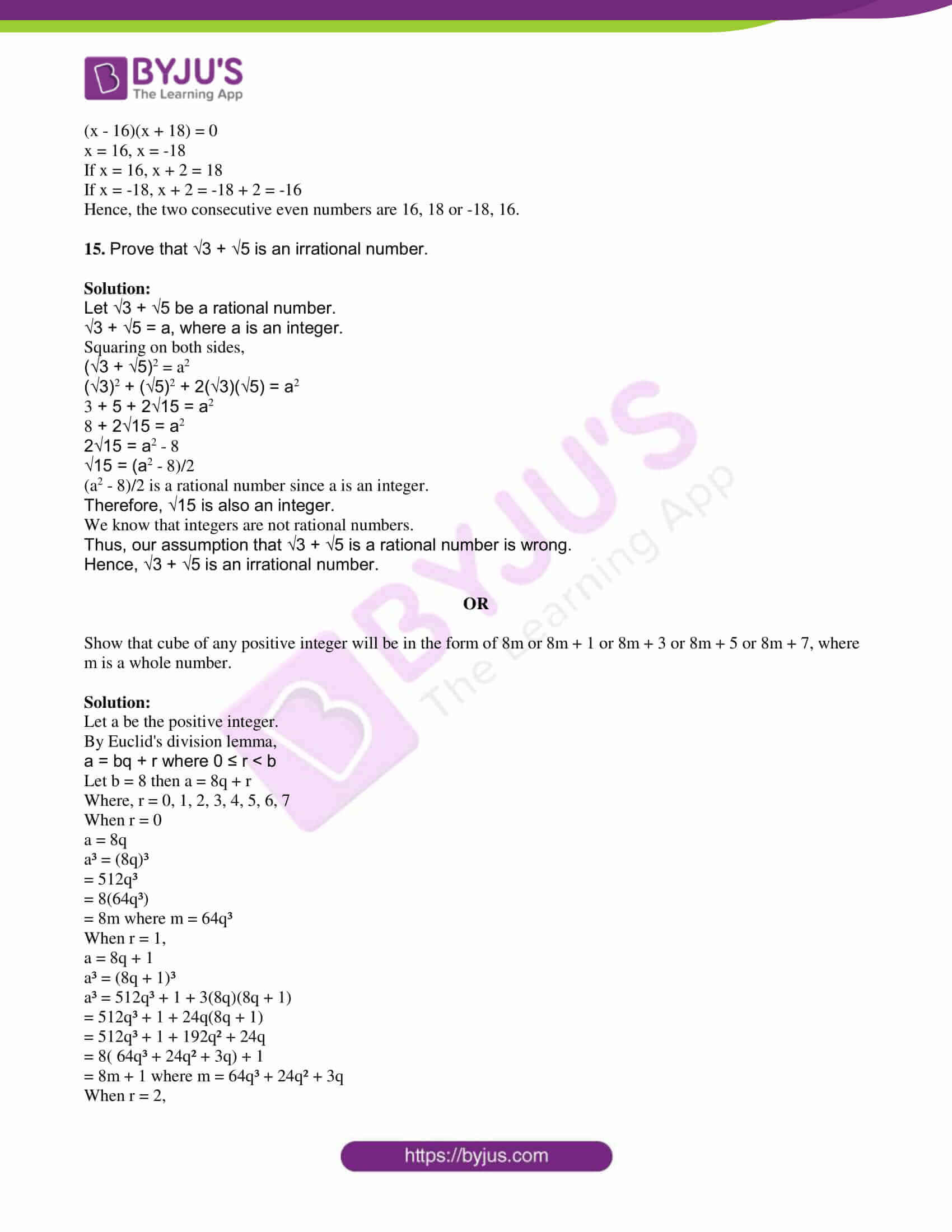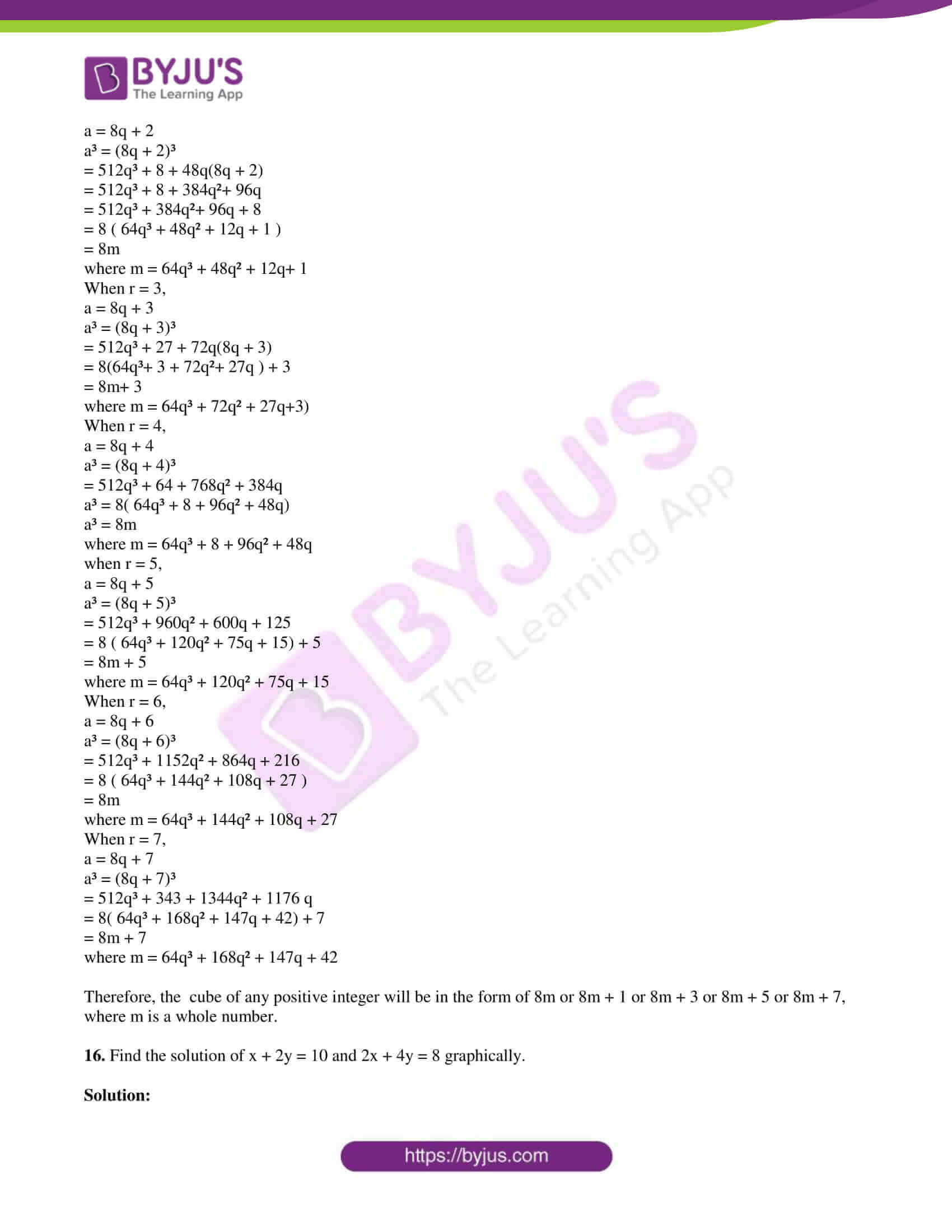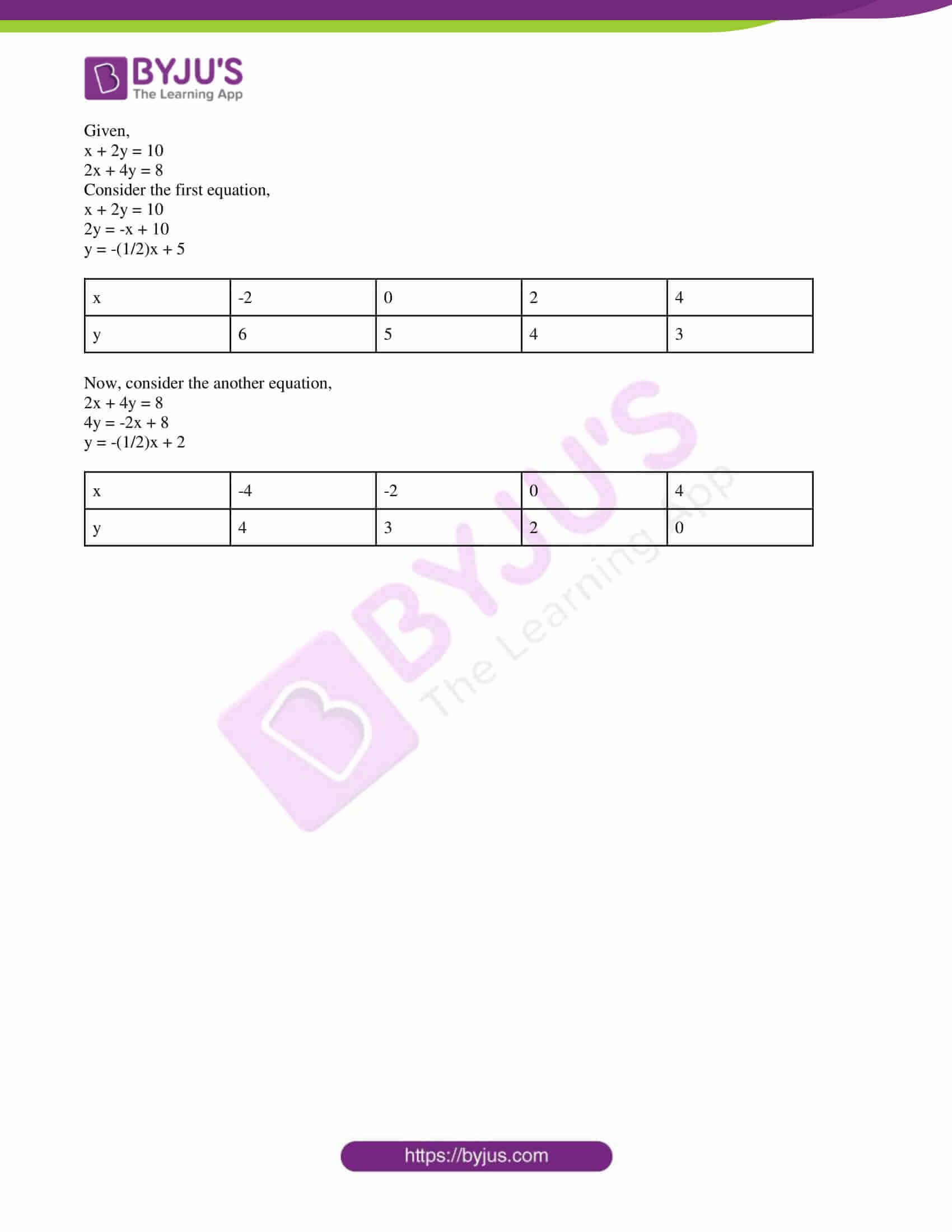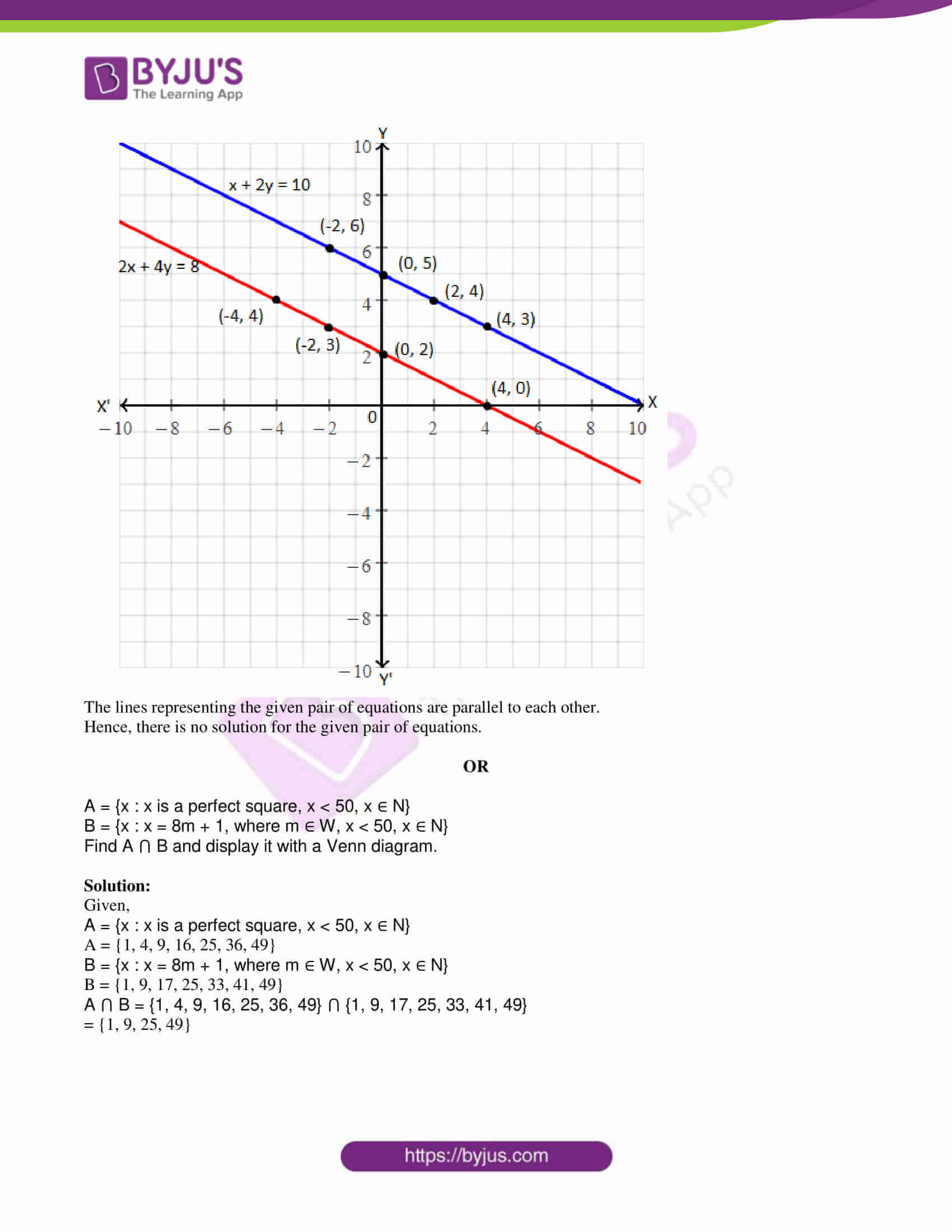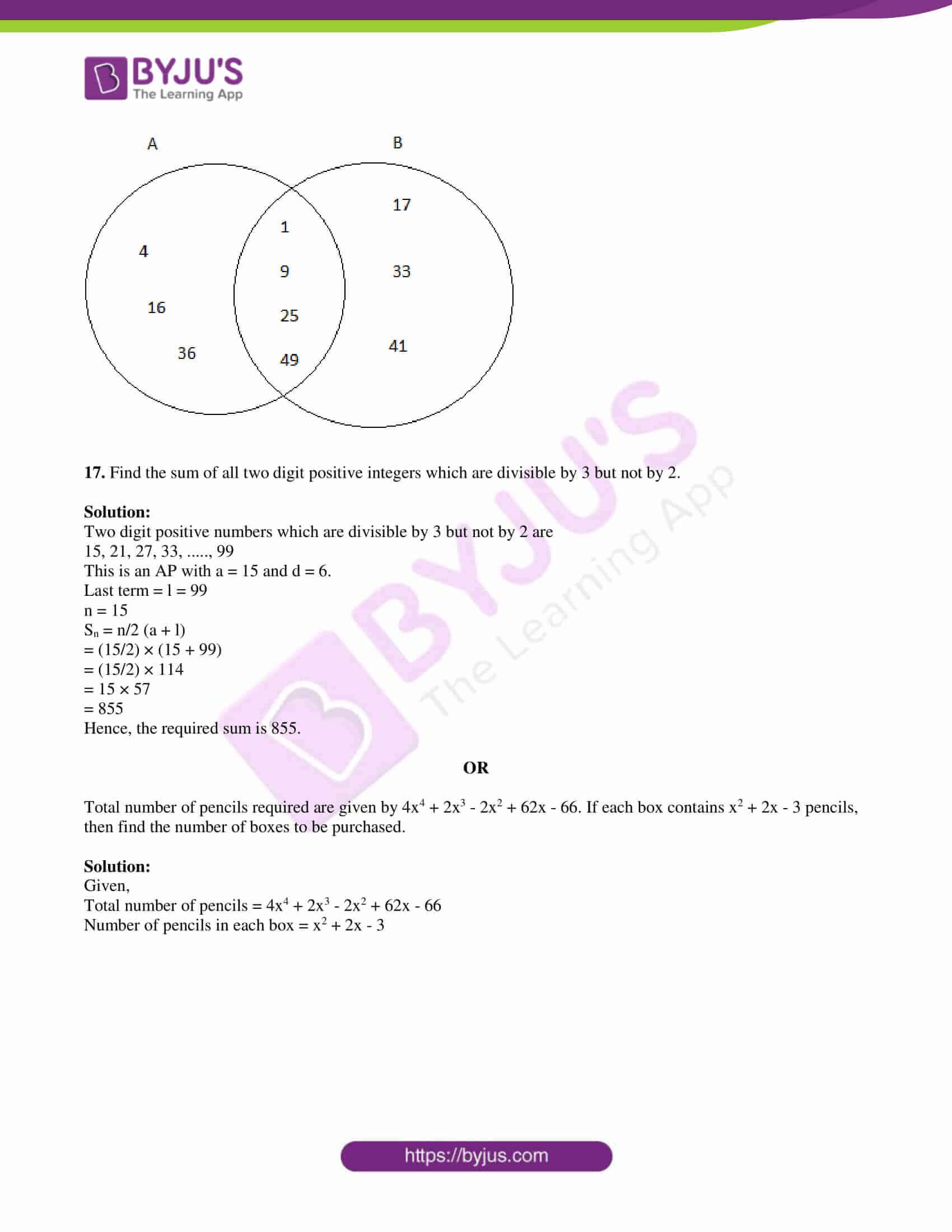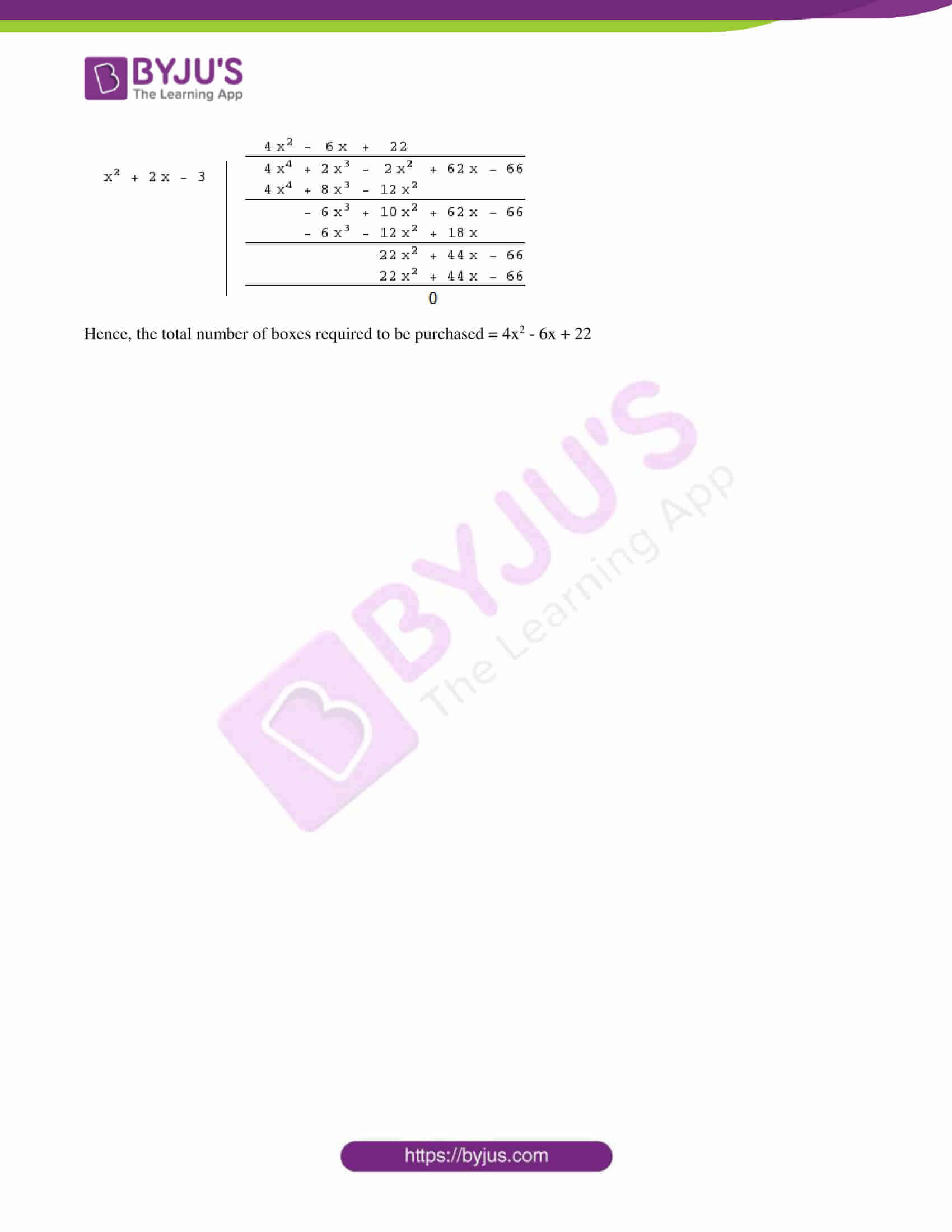PART A

SECTION – I

1. Find the distance between the points (1, 5) and (5, 8).

Solution:

Let the given points be:

(x1, y1) = (1, 5)

(x2, y2) = (5, 8)

Distance between two points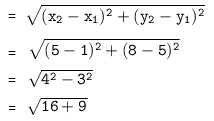= √25

= 5 units

2. Expand log10 385.

Solution:

log10 385

= log10 (5 × 7 × 11)

We know that log abc = log a + log b + log c

= log10 5 + log10 7 + log10 11

3. Give one example each for a finite set and an infinite set.

Solution:

Finite set: A = {3, 5, 7, 9, 11, 13, 15, 17}

n(A) = 8

Infinite set: Z = Set of all integers

= {…..,-4, -3, -2, -1, 0, 1, 2, 3, 4, …..}

n(Z) = ∞

4. Find the sum and product of roots of the quadratic equation.

x2 – 4√3x + 9 = 0

Solution:

x2 – 4√3x + 9 = 0

Comparing with the standard form ax2 + bx + c = 0,

a = 1, b = -4√3 and c = 9

Sum of the roots = -b/a = -(-4√3)/1 = 4√3

Product of the roots = c/a = 9/1 = 9

5. Is the sequence √3, √6, √9, √12,…. form an Arithmetic Progression? Give reason.

Solution:

Given,

√3, √6, √9, √12,…

First term = a = √3

Second term – First term = √6 – √3

Third term – Second term = √9 – √6 = 3 – √6

Since the common difference is not the same throughout the sequence, it is nor form an AP.

6. If x = a and y = b is the solution for the pair of equations x – y = 2 and x + y = 4, then find the values of a and b.

Solution:

Given pair of equations are:

x – y = 2….(i)

x + y = 4….(ii)

x – y + x + y = 2 + 4

2y = 6

y = 6/2 = 3

Substituting y = 3 in (i),

x – 3 = 2

x = 2 + 3

x = 5

Therefore, a = 5 and b = 3.

7. Verify the relation between zeroes and coefficients of the quadratic polynomial x2 – 4.

Solution:

Let the given quadratic polynomial p(x) = x2 – 4

Let p(x) = 0

x2 – 4 = 0

x2 = 4

x = √4

x = ±2

Therefore, zeroes of the given polynomial are -2 and 2.

Sum of the zeroes = -2 + 2 = 0 = -0/1 = -Coefficient of x/ Coefficient of x2

Product of the zeroes = (-2)(2) = -4 = -4/1 = Constant term/Coefficient of x2

Hence, verified the relationship between the zeroes and coefficients of the given quadratic polynomial.

SECTION – II

8. Complete the following table for the polynomial y = p(x) = x3 – 2x + 3.

 x -1 0 1 2 x3 -2x 3 y (x, y)

Solution:

Given,

y = p(x) = x3 – 2x + 3

 x -1 0 1 2 x3 -1 0 1 8 -2x 2 0 -2 -4 3 3 3 3 3 y 4 3 2 7 (x, y) (-1, 4) (0, 3) (1, 2) (2, 7)

9. Show that log (162/343) + 2 log(7/9) – log(1/7) = log 2

Solution:

LHS = log (162/343) + 2 log(7/9) – log(1/7)

= log (162/343) + log (7/9)2 – log (1/7)

= log (162/343) + log (49/81) – log (1/7)

= log [(162 × 49)/ (343 × 81)] – log (1/7)

= log (2/7) – log (1/7)

= log [(2/7)/ (1/7)]

= log 2

= RHS

Therefore, log (162/343) + 2 log(7/9) – log(1/7) = log 2

10. If the equation kx2 – 2kx + 6 = 0 has equal roots, then find the value of k.

Solution:

Given,

kx2 – 2kx + 6 = 0

Comparing with the standard form ax2 + bx + c = 0,

a = k, b = -2k, c = 6

Given that, the equation has equal roots.

⇒ b2 – 4ac = 0

⇒ (-2k)2 – 4(k)(6) = 0

⇒ 4k2 -24k = 0

⇒ 4k(k – 6) = 0

⇒ k(k – 6) = 0

⇒ k = 0, k = 6

k = 0 is not possible.

Hence, the value of k is 6.

11. Find the 7th term from the end of the arithmetic progression 7, 10, 13,…, 184.

Solution:

Given AP is:

7, 10, 13, …., 184

First term = a = 7

Common difference = d = 3

nth term of AP

an = a + (n – 1)d

a7 = 7 + (7 – 1)3

= 7 + (6) (3)

= 7 + 18

= 25

Therefore, the 7th term of the AP is 25.

12. In the diagram on a Lunar eclipse, if the positions of Sun, Earth and Moon are shown by (-4, 6), (k, -2) and (5, -6) respectively, then find the value of k.

Solution:

Given that the diagram on a Lunar eclipse, if the positions of Sun, Earth and Moon are shown by (-4, 6), (k, -2) and (5, -6) respectively.

That means the points lie on a straight line.

Hence, the area of the triangle formed by these points will be 0.

⇒ 1/2 [-4(-2 + 6) + k(-6 – 6) + 5(6 + 2)] = 0

⇒ -4(4) + k(-12) + 5(8) = 0

⇒ -16 – 12k + 40 = 0

⇒ 12k = 24

⇒ k = 24/12

⇒ k = 2

13. Given the linear equation 3x + 4y = 11, write linear equations in two variables such that their geometrical representations form parallel lines and intersecting lines.

Solution:

Given,

Equation of first line is 3x + 4y = 11

Let ax + by + c be the equation of the second line.

Condition for parallel lines:

a1/a2 = b1/b2 ≠ c1/c2

3/a = 4/b ≠ 11/c

Let us consider some values of a, b, c which satisfies the above condition.

a = 6, b = 8, c = 14

Therefore, the equation of line which is parallel to the given line is 6x + 8y + 14 = 0

Condition for intersecting lines:

a1/a2 ≠ b1/b2

3/a ≠ 4/b

Let us consider some values of a, b, c which satisfies the above condition.

a = 8, b = 6, c = 11

8x + 6y + 11 = 0

Therefore, the equation of line which is perpendicular to the given line is 8x + 6y + 11 = 0

SECTION – III

14. Find the points of trisection of the line segment joining the points (-2, 1) and (7, 4).

Solution:

Let the given points be:

A = (x1, y1) = (-2, 1)

B = (x2, y2) = (7, 4)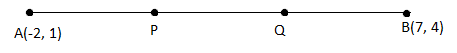P divides AB in the ratio 1 : 2.

Here, m : n = 1 : 2

Using the section formula,

P(x, y) = [(mx2 + nx1)/ (m + n), (my2 + ny1)/ (m + n)]

= [(7 – 4)/ (1 + 2), (4 + 2)/ (1 + 2)]

= (3/3, 6/3)

= (1, 2)

Q is the midpoint of PB.

Q = [(1 + 7)/2, (2 + 4)/3]

= (8/2, 6/2)

= (4, 3)

Hence, the required points are (1, 2) and (4, 3).

OR

Sum of squares of two consecutive even numbers is 580. Find the numbers by writing a suitable quadratic equation.

Solution:

Let x and (x + 2) be the two consecutive even numbers.

According to the given,

x2 + (x + 2)2 = 580

x2 + x2 + 4x + 4 – 580 = 0

2x2 + 4x – 576 = 0

2(x2 + 2x – 288) = 0

x2 + 2x – 288 = 0

x2 + 18x – 16x – 288 = 0

x(x + 18) – 16(x + 18) = 0

(x – 16)(x + 18) = 0

x = 16, x = -18

If x = 16, x + 2 = 18

If x = -18, x + 2 = -18 + 2 = -16

Hence, the two consecutive even numbers are 16, 18 or -18, 16.

15. Prove that √3 + √5 is an irrational number.

Solution:

Let √3 + √5 be a rational number.

√3 + √5 = a, where a is an integer.

Squaring on both sides,

(√3 + √5)2 = a2

(√3)2 + (√5)2 + 2(√3)(√5) = a2

3 + 5 + 2√15 = a2

8 + 2√15 = a2

2√15 = a2 – 8

√15 = (a2 – 8)/2

(a2 – 8)/2 is a rational number since a is an integer.

Therefore, √15 is also an integer.

We know that integers are not rational numbers.

Thus, our assumption that √3 + √5 is a rational number is wrong.

Hence, √3 + √5 is an irrational number.

OR

Show that cube of any positive integer will be in the form of 8m or 8m + 1 or 8m + 3 or 8m + 5 or 8m + 7, where m is a whole number.

Solution:

Let a be the positive integer.

By Euclid’s division lemma,

a = bq + r where 0 ≤ r < b

Let b = 8 then a = 8q + r

Where, r = 0, 1, 2, 3, 4, 5, 6, 7

When r = 0

a = 8q

a³ = (8q)³

= 512q³

= 8(64q³)

= 8m where m = 64q³

When r = 1,

a = 8q + 1

a³ = (8q + 1)³

a³ = 512q³ + 1 + 3(8q)(8q + 1)

= 512q³ + 1 + 24q(8q + 1)

= 512q³ + 1 + 192q² + 24q

= 8( 64q³ + 24q² + 3q) + 1

= 8m + 1 where m = 64q³ + 24q² + 3q

When r = 2,

a = 8q + 2

a³ = (8q + 2)³

= 512q³ + 8 + 48q(8q + 2)

= 512q³ + 8 + 384q²+ 96q

= 512q³ + 384q²+ 96q + 8

= 8 ( 64q³ + 48q² + 12q + 1 )

= 8m

where m = 64q³ + 48q² + 12q+ 1

When r = 3,

a = 8q + 3

a³ = (8q + 3)³

= 512q³ + 27 + 72q(8q + 3)

= 8(64q³+ 3 + 72q²+ 27q ) + 3

= 8m+ 3

where m = 64q³ + 72q² + 27q+3)

When r = 4,

a = 8q + 4

a³ = (8q + 4)³

= 512q³ + 64 + 768q² + 384q

a³ = 8( 64q³ + 8 + 96q² + 48q)

a³ = 8m

where m = 64q³ + 8 + 96q² + 48q

when r = 5,

a = 8q + 5

a³ = (8q + 5)³

= 512q³ + 960q² + 600q + 125

= 8 ( 64q³ + 120q² + 75q + 15) + 5

= 8m + 5

where m = 64q³ + 120q² + 75q + 15

When r = 6,

a = 8q + 6

a³ = (8q + 6)³

= 512q³ + 1152q² + 864q + 216

= 8 ( 64q³ + 144q² + 108q + 27 )

= 8m

where m = 64q³ + 144q² + 108q + 27

When r = 7,

a = 8q + 7

a³ = (8q + 7)³

= 512q³ + 343 + 1344q² + 1176 q

= 8( 64q³ + 168q² + 147q + 42) + 7

= 8m + 7

where m = 64q³ + 168q² + 147q + 42

Therefore, the cube of any positive integer will be in the form of 8m or 8m + 1 or 8m + 3 or 8m + 5 or 8m + 7, where m is a whole number.

16. Find the solution of x + 2y = 10 and 2x + 4y = 8 graphically.

Solution:

Given,

x + 2y = 10

2x + 4y = 8

Consider the first equation,

x + 2y = 10

2y = -x + 10

y = -(1/2)x + 5

 x -2 0 2 4 y 6 5 4 3

Now, consider the another equation,

2x + 4y = 8

4y = -2x + 8

y = -(1/2)x + 2

 x -4 -2 0 4 y 4 3 2 0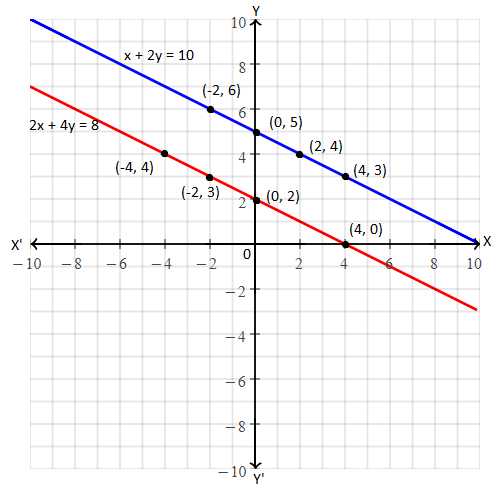The lines representing the given pair of equations are parallel to each other.

Hence, there is no solution for the given pair of equations.

OR

A = {x : x is a perfect square, x < 50, x ∈ N}

B = {x : x = 8m + 1, where m ∈ W, x < 50, x ∈ N}

Find A ⋂ B and display it with a Venn diagram.

Solution:

Given,

A = {x : x is a perfect square, x < 50, x ∈ N}

A = {1, 4, 9, 16, 25, 36, 49}

B = {x : x = 8m + 1, where m ∈ W, x < 50, x ∈ N}

B = {1, 9, 17, 25, 33, 41, 49}

A ⋂ B = {1, 4, 9, 16, 25, 36, 49} ⋂ {1, 9, 17, 25, 33, 41, 49}

= {1, 9, 25, 49}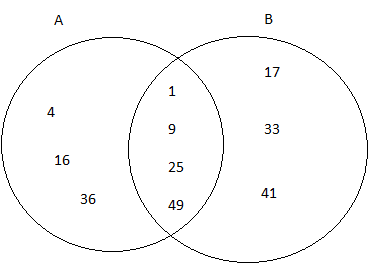17. Find the sum of all two digit positive integers which are divisible by 3 but not by 2.

Solution:

Two digit positive numbers which are divisible by 3 but not by 2 are

15, 21, 27, 33, ….., 99

This is an AP with a = 15 and d = 6.

Last term = l = 99

n = 15

Sn = n/2 (a + l)

= (15/2) × (15 + 99)

= (15/2) × 114

= 15 × 57

= 855

Hence, the required sum is 855.

OR

Total number of pencils required are given by 4x4 + 2x3 – 2x2 + 62x – 66. If each box contains x2 + 2x – 3 pencils, then find the number of boxes to be purchased.

Solution:

Given,

Total number of pencils = 4x4 + 2x3 – 2x2 + 62x – 66

Number of pencils in each box = x2 + 2x – 3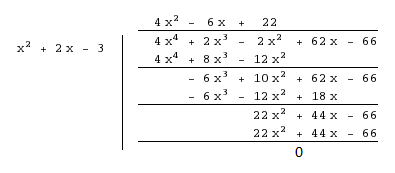Hence, the total number of boxes required to be purchased = 4x2 – 6x + 22